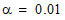# NMath User's Guide

45.2 Two Sample Kolmogorov-Smirnov Test (.NET, C#, CSharp, VB, Visual Basic, F#)

Class performs a two-sample Kolmogorov-Smirnov test to compare the distributions of values in two data sets. For each potential value x, the Kolmogorov-Smirnov test compares the proportion of values in the first sample less than x with the proportion of values in the second sample less than x. The null hypothesis is that the two samples have the same continuous distribution. The alternative hypothesis is that they have different continuous distributions.

Sample data can be passed to the constructor as vectors, numeric columns in a data frame, or arrays of doubles. Thus:

Code Example – C# Kolmogorov-Smirnov test

```var ks = new TwoSampleKSTest( data1, data2 );
```

Code Example – VB Kolmogorov-Smirnov test

```Dim KS As New TwoSampleKSTest(Data1, Data2)
```

By default, a TwoSampleKSTest object performs the Kolmogorov-Smirnov test with. A different alpha level can be specified at the time of construction using constructor overloads, or after construction using the provided Alpha property.

Once you've constructed and configured a TwoSampleKSTest object, you can access the various test results using the provided properties:

Code Example – C# Kolmogorov-Smirnov test

```Console.WriteLine( "statistic = " + test.Statistic );
```
```Console.WriteLine( "p-value = " + test.P );
```
```Console.WriteLine( "alpha = " + test.Alpha );
```
```Console.WriteLine( "reject the null hypothesis? " + test.Reject);
```

Code Example – VB Kolmogorov-Smirnov test

```Console.WriteLine("statistic = " & Test.Statistic)
```
```Console.WriteLine("p-value = " & Test.P)
```
```Console.WriteLine("alpha = " & Test.Alpha)
```
```Console.WriteLine("reject the null hypothesis? " & Test.Reject)
```

Top

Top